• 统计机器学习的策略通常是最小化代价函数(目标函数)，因此代价函数的选取对学习效果影响很大。损失函数与代价函数是一回事，但有的书籍定义损失函数指单个样本的损失(lll),而代价函数指损失函数之和或加上正则化项...
统计机器学习的策略通常是最小化代价函数(目标函数)，因此代价函数的选取对学习效果影响很大。损失函数与代价函数是一回事，但有的书籍定义损失函数指单个样本的损失($l$),而代价函数指损失函数之和或加上正则化项($L$)。本文用$l$表示单个样本的损失，$L$表示所有样本的平均损失，$y^p$表示预测值$f(x)$。
分类模型损失函数
分类模型的损失函数通常用$yy^p$来表示。
Zero-One
0-1损失函数对每个错分类点施加相同的惩罚。表达式如下：l(y_i, y_i^p)=\left\{ \begin{aligned} 0 \qquad y_iy_i^p\ge 0 \\ 1\qquad y_iy_i^p\lt 0\end{aligned} \right.
Logarithmic
对数损失函数的形式是：$l(y_i, y_i^p)=-logP(y|x)$最小化对数损失函数与极大对数形式的似然函数是等价得。
Hinge
支持向量机采用的损失函数。表达式如下：$l(y_i, y_i^p)=max(0, 1-y_iy_i^p)$可见该损失函数使得$y_iy_i^p$大于1的样本损失值为0，抛弃其在模型构建中的作用，即非支持向量对模型的构建不产生任何作用。
Exponential
指数损失是Adaboost中的损失函数，表达式如下：$l(y_i, y_i^p)=e^{-y_iy_i^p}$预测结果与真实值相差较大时，损失值过大，对局外点过于敏感。
Modified Huber
Huber损失函数在分类模型中的变种，结合了Hinge以及Logistic损失函数的优点。表达式如下：l_{\delta}(y_i,y_i^p)=\left\{ \begin{aligned} max(0, 1-y_iy_i^p)^2 \qquad y_iy_i^p|\ge-1 \\ -4y_iy_i^p\qquad otherwise \end{aligned} \right.可见在$y_iy_i^p \ge 1$时产生稀疏解提高训练效率,对预测误差较大的样本($y_iy_i^p \lt -1$)，惩罚以线性增加，受局外点的影响较小。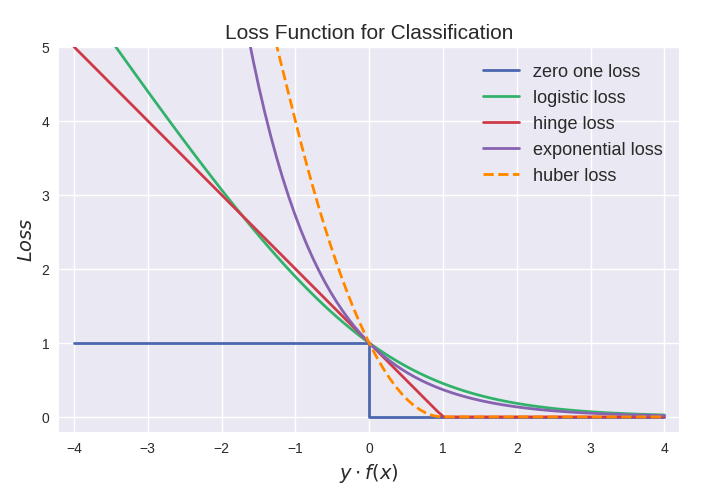回归模型损失函数
MSE
平方误差损失函数(mean square error,MSE )或称quadratic loss、L2损失函数，是最常用的损失函数。表达式如下：$L(y,y^p)=\frac{\sum_{i=1}^{n}{(y_i-y_i^p)^2}}{n}$
优点：曲线平滑，一阶二阶均可导，在极值点导数也不会有很大跃变
缺点：对于局外点，其值过大，导致模型会过分拟合局外点，性能降低
MAE
平均绝对误差损失函数(mean absolute error, MAE)，又称L1损失函数。表达式如下：$L(y,y^p)=\frac{\sum_{i=1}^{n}{|y_i-y_i^p|}}{n}$
优点：对局外点不像MSE那么敏感
缺点：在极值点梯度跃变(不可导)，靠近极值点时需要降低学习率
Huber
Huber损失函数可以看作MSE以及MAE的结合。表达式如下：l_{\delta}(y_i,y_i^p)=\left\{ \begin{aligned} \frac12(y_i -y_i^p)^2 \qquad |y_i-y_i^p|\le\delta \\ \delta |y_i-y_i^p| - \frac 12\delta^2\qquad otherwise \end{aligned} \right.
可见$\delta$趋近于0则退化成MAE, 趋于无穷则退化成MSE。
优点： 综合了MSE以及MAE的优点，即既对局外点不敏感，同时连续可导。
缺点：超参数$\delta$不容易调节
Log-Cosh
对数双曲余弦损失函数是一种比MSE更加光滑的损失函数。表达式如下：$L(y,y^p)=\sum_{i=1}^{n}{log(cosh(y_i^p-y_i))}$
优点：拥有Huber损失函数的所有优点，且处处二阶可导
缺点：当预测值与真实值相差非常大时，一阶二阶导数接近于常数
Quantile
分位数损失函数通过分位数$\gamma$对过拟合以及欠拟合施加不同的权重。$\gamma>0.5$惩罚过拟合较多，反之惩罚欠拟合较多。$L_{\gamma}(y, y^p)=\sum_{i=y_i<y_i^p}{(\gamma -1)|y_i-y_i^p|+\sum_{i=y_i\ge y_i^p}{\gamma|y_i-y_i^p|}}$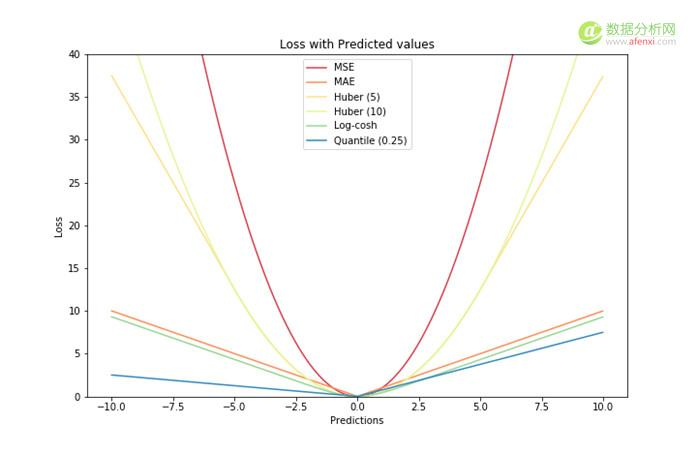深度学习模型损失函数
深度学习模型通常用交叉熵(cross entropy)损失函数，可以很好地衡量真实分布与输出分布之间的差距。令真实分布为$P(X)$，模型输出分布为$Q(X)$，则交叉熵为$H(P, Q)=-\sum_i{P(i)logQ(i)}$
参考资料
https://www.cnblogs.com/massquantity/p/8964029.html
https://www.afenxi.com/56326.html
https://en.wikipedia.org/wiki/Huber_loss
注：如有不当之处，请指正。


展开全文• 大家好，最近有朋友留言询问为什么在机器学习中损失函数用2n为底，如下公式所示。损失函数如上图所示，MSE(Mean-square error，均方误差)是比较常用的损失函数方程。确实，MSE公式中，如果使用n替换掉2n，可以达到...
大家好，最近有朋友留言询问为什么在机器学习中的损失函数用2n为底，如下公式所示。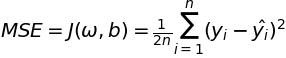损失函数如上图所示，MSE(Mean-square error，均方误差)是比较常用的损失函数方程。确实，MSE公式中，如果使用n替换掉2n，可以达到同样的效果。那么，为什么大部分公式中倾向保留2n呢？机器学习的目的在机器学习中，当有了数据集(dataset)，我们需要创建一个模型，使得模型的预测值和数据集中的真实值尽可能地接近。但是，预测值和真实值是几乎不可能一样的，这样就存在一个误差值。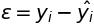误差其中，机器学习的目的是不停地迭代，使得模型的预测值和数据集中的真实值的总体误差尽量小。误差的分布假设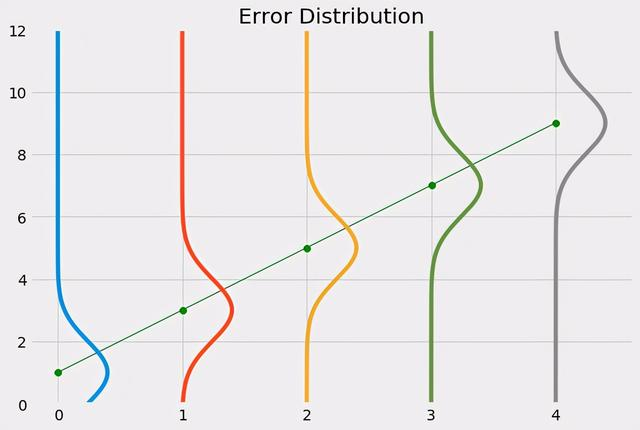误差分布也就是说，当我们使用机器学习模型的时候，我们是允许误差的存在。简单来说，训练数据集中有多少个数据点，就会生成相应数量的误差。简单来说，我们可以假设所有的误差符合一个统计分布，比如正态分布。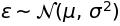误差的正态分布对于误差来说，自然希望误差尽可能接近0。这样可以假设误差符合标准正态分布。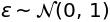误差的标准正态分布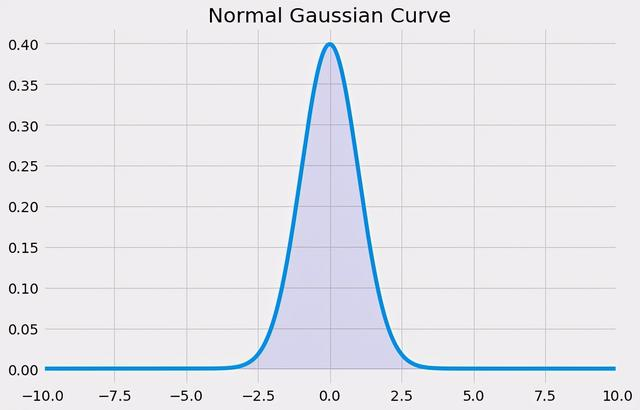标准正态分布通过标准正态分布的公式可以得到误差的分布公式，如下：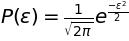误差的标准正态分布公式对上式左右取对数，可得：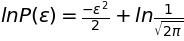上式只是对于数据集中的一个数据点的计算，当我们需要求出所有数据点的平均值的时候，可以得到MSE的计算公式，当然第二项是常数项，在计算中可以省略。损失函数求导数方便当然，使用2n而不是n对于之后的损失函数求导也会方便很多。由于(x^2)' = 2x。这样一来，求导之后，2会被约分掉。总结很多时候，公式里的常数项会有一定的理由存在。不用去死记硬背公式，我们需要的是去理解公式本身的含义。
展开全文• Log Loss 在使用似然函数最大化时，其形式是进行连乘，但是...因此log类型的损失函数也是一种常见的损失函数． 一些常用的损失函数： (1) Zero-one loss (2) Perceptron loss (3) Hinge loss (4) Log loss (5) S
Log Loss 在使用似然函数最大化时，其形式是进行连乘，但是为了便于处理，一般会套上log，这样便可以将连乘转化为求和，由于log函数是单调递增函数，因此不会改变优化结果。因此log类型的损失函数也是一种常见的损失函数．
一些常用的损失函数：
(1) Zero-one loss
(2) Perceptron loss
(3) Hinge loss
(4) Log loss
(5) Square loss
(6) Absolute loss
(7) Exponential loss
展开全文• 大家好，最近有朋友留言询问为什么在机器学习中损失函数用2n为底，如下公式所示。损失函数如上图所示，MSE(Mean-square error，均方误差)是比较常用的损失函数方程。确实，MSE公式中，如果使用n替换掉2n，可以达到...
大家好，最近有朋友留言询问为什么在机器学习中的损失函数用2n为底，如下公式所示。损失函数如上图所示，MSE(Mean-square error，均方误差)是比较常用的损失函数方程。确实，MSE公式中，如果使用n替换掉2n，可以达到同样的效果。那么，为什么大部分公式中倾向保留2n呢？机器学习的目的在机器学习中，当有了数据集(dataset)，我们需要创建一个模型，使得模型的预测值和数据集中的真实值尽可能地接近。但是，预测值和真实值是几乎不可能一样的，这样就存在一个误差值。误差其中，机器学习的目的是不停地迭代，使得模型的预测值和数据集中的真实值的总体误差尽量小。误差的分布假设误差分布也就是说，当我们使用机器学习模型的时候，我们是允许误差的存在。简单来说，训练数据集中有多少个数据点，就会生成相应数量的误差。简单来说，我们可以假设所有的误差符合一个统计分布，比如正态分布。误差的正态分布对于误差来说，自然希望误差尽可能接近0。这样可以假设误差符合标准正态分布。误差的标准正态分布标准正态分布通过标准正态分布的公式可以得到误差的分布公式，如下：误差的标准正态分布公式对上式左右取对数，可得：上式只是对于数据集中的一个数据点的计算，当我们需要求出所有数据点的平均值的时候，可以得到MSE的计算公式，当然第二项是常数项，在计算中可以省略。损失函数求导数方便当然，使用2n而不是n对于之后的损失函数求导也会方便很多。由于(x^2)' = 2x。这样一来，求导之后，2会被约分掉。总结很多时候，公式里的常数项会有一定的理由存在。不用去死记硬背公式，我们需要的是去理解公式本身的含义。
展开全文...